Limits: Derivatives of Polynomials
 Home > Lessons > Limits: Derivatives of Polynomials Search | Updated May 28th, 2021
Introduction

This lesson page will inform you how to find a derivative of a function using the definition of a derivative. Here are the sections within this page:

In order to understand derivatives, you must understand several concepts and skills. Review any of these lessons in order to prepare for derivatives.ideo: Calculating Slopeesson: Functionsesson: Limits

 Description of a Derivative The derivative of a function refers to the slope of a tangent line of a function at a certain point. Here is a graphical representation of a tangent line for three different parabolas.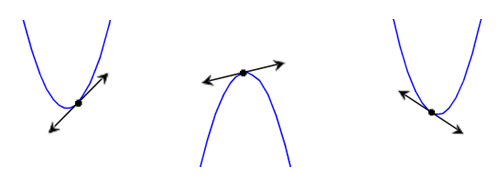To fully understand derivatives, we need to look at its definition. Definition of a Derivative The definition of a derivative is a blend of pre-calculus and algebra. Here it is for any function f(x).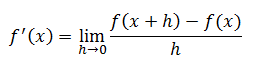If you would like to learn where this definition comes from, watch this video.ideo: Derivation of a Derivative Example 1 Using the definition of a derivative, find the derivative of f(x), given below.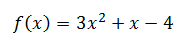To get started we write the definition of a derivative.Now, we substitute x + h into the function f(x) and subtract f(x) from it.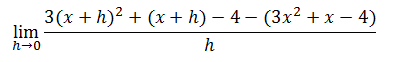Notice how parentheses were used for substitution. This is extremely important.     Also notice what happens if we were to allow the h-value to go to zero.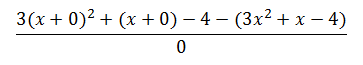We would have a zero in the denominator, which is illogical. So, we have to employ other – more algebraic – methods for finding this limit.     Let’s square the x + h, using algebra instead of letting h go to zero.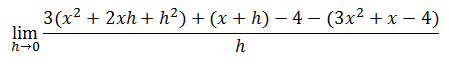Next we need to drop the parentheses and use the distributive property, where necessary.We can simplify the numerator by cleaning up terms.Next, we can simplify the numerator by using algebra.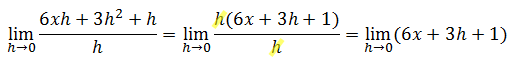Since we no longer have h in a denominator, let h go to zero.This means…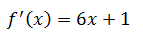It means the slope of the tangent line is a function dependent upon the location of the point on the function. The slope of the tangent line changes depending on where we look on the function.     If we were interested in finding the slope of the tangent line at x = 3, we would do this…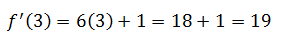Here is the function (in blue) and the tangent line (in black) at x = 3.If we wanted to know the slope of the tangent line at x = -1, we would do this…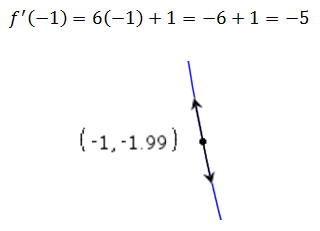ideo: Derivative of a Polynomialuiz: Derivatives of Polynomials Example 2 Let’s find the derivative of this function using the definition of a derivative.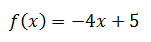Here is the definition of a derivative.We need to substitute x + h into the function and subtract the function from it.Next, use the distributive property to drop the parentheses.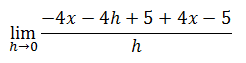We can clean up some terms.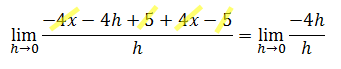Next, simplify the expression.Allowing h to go to zero is peculiar for this problem because there are no more h’s that remain. So, allowing h to go to zero does not change the result. The result is simply -4.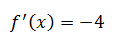This may seem strange until we look at the original function, f(x).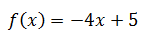The function f(x) is a line. It has a y-intercept of 5 and a slope of -4. That means everywhere along the line the line has a slope of -4. This is exactly what the derivative is telling us. The derivative tells us the slope of the tangent line is -4, regardless of where we look on the line. Here is the graph of f(x) and a tangent line to the function.ideo: Derivative of a Polynomialuiz: Derivatives of Polynomials Instructional Videos Try this instructional video to learn this lesson.ideo: Derivative of a Polynomial Interactive Quizmasters Try this interactive quizmaster to learn this lesson.uiz: Derivatives of Polynomials Related Lessons Try these lessons, which are related to the sections above.esson: Limitsesson: Limits by Factoringesson: Continuity